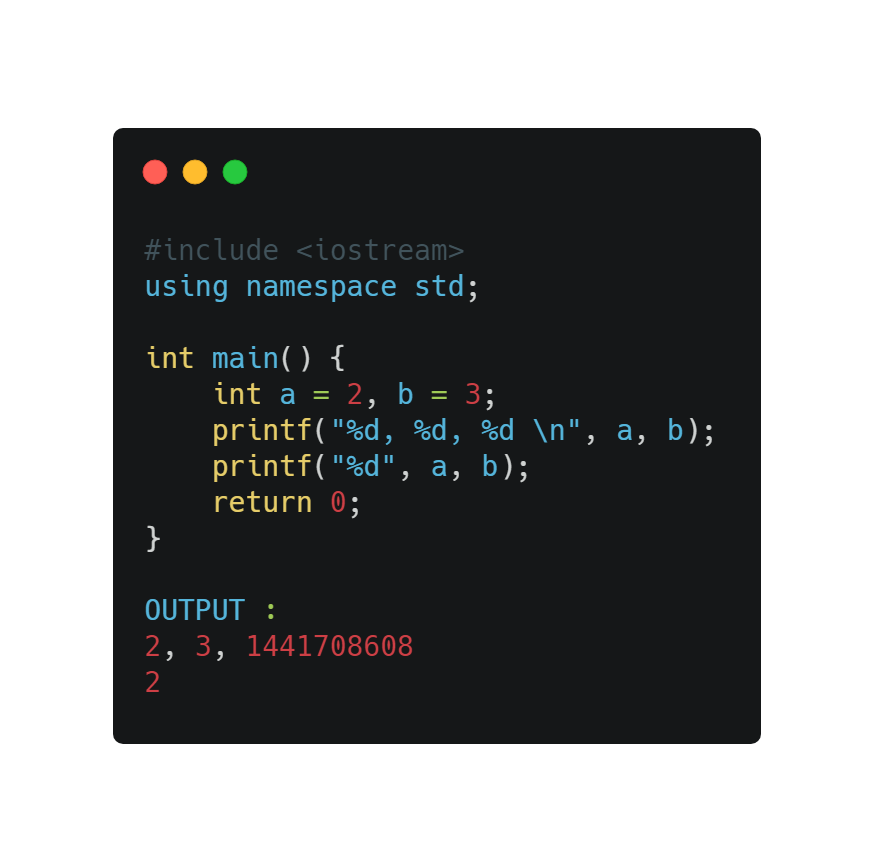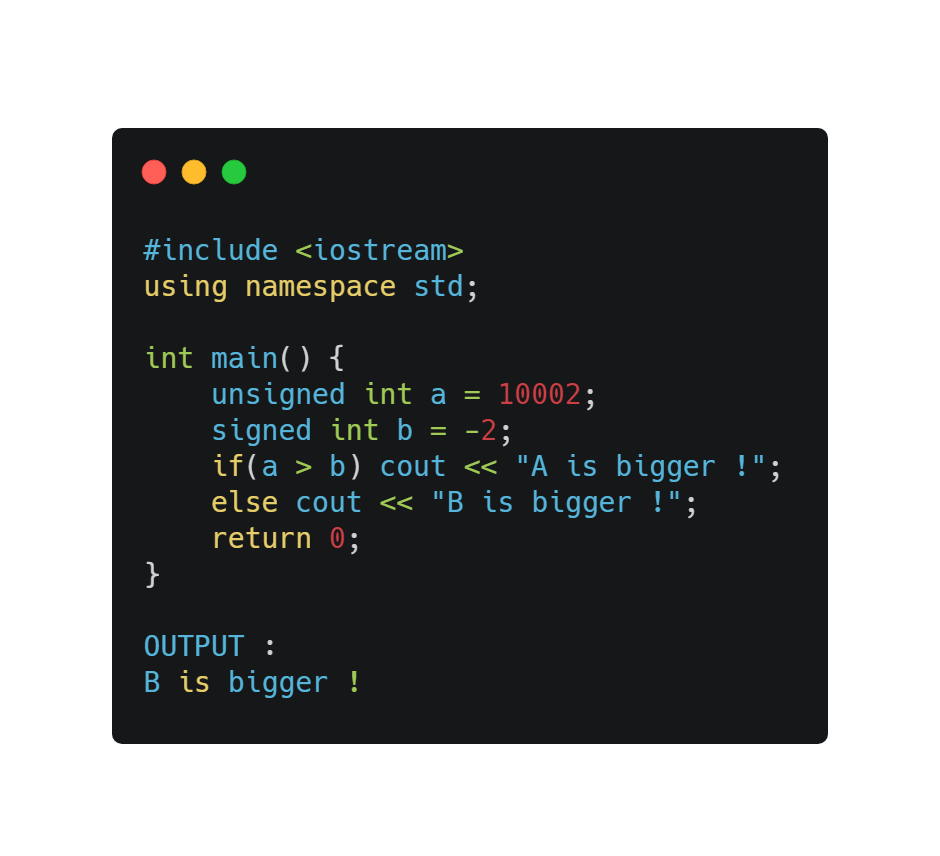1651546800

# Klib | A Standalone and Lightweight C Library

Klib is a standalone and lightweight C library distributed under MIT/X11 license. Most components are independent of external libraries, except the standard C library, and independent of each other. To use a component of this library, you only need to copy a couple of files to your source code tree without worrying about library dependencies.

Klib strives for efficiency and a small memory footprint. Some components, such as khash.h, kbtree.h, ksort.h and kvec.h, are among the most efficient implementations of similar algorithms or data structures in all programming languages, in terms of both speed and memory use.

A new documentation is available here which includes most information in this README file.

## Methodology

For the implementation of generic containers, klib extensively uses C macros. To use these data structures, we usually need to instantiate methods by expanding a long macro. This makes the source code look unusual or even ugly and adds difficulty to debugging. Unfortunately, for efficient generic programming in C that lacks template, using macros is the only solution. Only with macros, we can write a generic container which, once instantiated, compete with a type-specific container in efficiency. Some generic libraries in C, such as Glib, use the `void*` type to implement containers. These implementations are usually slower and use more memory than klib (see this benchmark).

To effectively use klib, it is important to understand how it achieves generic programming. We will use the hash table library as an example:

``````#include "khash.h"
KHASH_MAP_INIT_INT(m32, char)        // instantiate structs and methods
int main() {
int ret, is_missing;
khint_t k;
khash_t(m32) *h = kh_init(m32);  // allocate a hash table
k = kh_put(m32, h, 5, &ret);     // insert a key to the hash table
if (!ret) kh_del(m32, h, k);
kh_value(h, k) = 10;             // set the value
k = kh_get(m32, h, 10);          // query the hash table
is_missing = (k == kh_end(h));   // test if the key is present
k = kh_get(m32, h, 5);
kh_del(m32, h, k);               // remove a key-value pair
for (k = kh_begin(h); k != kh_end(h); ++k)  // traverse
if (kh_exist(h, k))          // test if a bucket contains data
kh_value(h, k) = 1;
kh_destroy(m32, h);              // deallocate the hash table
return 0;
}
``````

In this example, the second line instantiates a hash table with `unsigned` as the key type and `char` as the value type. `m32` names such a type of hash table. All types and functions associated with this name are macros, which will be explained later. Macro `kh_init()` initiates a hash table and `kh_destroy()` frees it. `kh_put()` inserts a key and returns the iterator (or the position) in the hash table. `kh_get()` and `kh_del()` get a key and delete an element, respectively. Macro `kh_exist()` tests if an iterator (or a position) is filled with data.

An immediate question is this piece of code does not look like a valid C program (e.g. lacking semicolon, assignment to an apparent function call and apparent undefined `m32` 'variable'). To understand why the code is correct, let's go a bit further into the source code of `khash.h`, whose skeleton looks like:

``````#define KHASH_INIT(name, SCOPE, key_t, val_t, is_map, _hashf, _hasheq) \
typedef struct { \
int n_buckets, size, n_occupied, upper_bound; \
unsigned *flags; \
key_t *keys; \
val_t *vals; \
} kh_##name##_t; \
SCOPE inline kh_##name##_t *init_##name() { \
return (kh_##name##_t*)calloc(1, sizeof(kh_##name##_t)); \
} \
SCOPE inline int get_##name(kh_##name##_t *h, key_t k) \
... \
SCOPE inline void destroy_##name(kh_##name##_t *h) { \
if (h) { \
free(h->keys); free(h->flags); free(h->vals); free(h); \
} \
}

#define _int_hf(key) (unsigned)(key)
#define _int_heq(a, b) (a == b)
#define khash_t(name) kh_##name##_t
#define kh_value(h, k) ((h)->vals[k])
#define kh_begin(h, k) 0
#define kh_end(h) ((h)->n_buckets)
#define kh_init(name) init_##name()
#define kh_get(name, h, k) get_##name(h, k)
#define kh_destroy(name, h) destroy_##name(h)
...
#define KHASH_MAP_INIT_INT(name, val_t) \
KHASH_INIT(name, static, unsigned, val_t, is_map, _int_hf, _int_heq)
``````

`KHASH_INIT()` is a huge macro defining all the structs and methods. When this macro is called, all the code inside it will be inserted by the C preprocess to the place where it is called. If the macro is called multiple times, multiple copies of the code will be inserted. To avoid naming conflict of hash tables with different key-value types, the library uses token concatenation, which is a preprocessor feature whereby we can substitute part of a symbol based on the parameter of the macro. In the end, the C preprocessor will generate the following code and feed it to the compiler (macro `kh_exist(h,k)` is a little complex and not expanded for simplicity):

``````typedef struct {
int n_buckets, size, n_occupied, upper_bound;
unsigned *flags;
unsigned *keys;
char *vals;
} kh_m32_t;
static inline kh_m32_t *init_m32() {
return (kh_m32_t*)calloc(1, sizeof(kh_m32_t));
}
static inline int get_m32(kh_m32_t *h, unsigned k)
...
static inline void destroy_m32(kh_m32_t *h) {
if (h) {
free(h->keys); free(h->flags); free(h->vals); free(h);
}
}

int main() {
int ret, is_missing;
khint_t k;
kh_m32_t *h = init_m32();
k = put_m32(h, 5, &ret);
if (!ret) del_m32(h, k);
h->vals[k] = 10;
k = get_m32(h, 10);
is_missing = (k == h->n_buckets);
k = get_m32(h, 5);
del_m32(h, k);
for (k = 0; k != h->n_buckets; ++k)
if (kh_exist(h, k)) h->vals[k] = 1;
destroy_m32(h);
return 0;
}
``````

This is the C program we know.

From this example, we can see that macros and the C preprocessor plays a key role in klib. Klib is fast partly because the compiler knows the key-value type at the compile time and is able to optimize the code to the same level as type-specific code. A generic library written with `void*` will not get such performance boost.

Massively inserting code upon instantiation may remind us of C++'s slow compiling speed and huge binary size when STL/boost is in use. Klib is much better in this respect due to its small code size and component independency. Inserting several hundreds lines of code won't make compiling obviously slower.

## Resources

• Library documentation, if present, is available in the header files. Examples can be found in the test/ directory.
• Obsolete documentation of the hash table library can be found at SourceForge. This README is partly adapted from the old documentation.
• Blog post describing the hash table library.
• Blog post on why using `void*` for generic programming may be inefficient.
• Blog post on the generic stream buffer.
• Blog post evaluating the performance of `kvec.h`.
• Blog post arguing B-tree may be a better data structure than a binary search tree.
• Blog post evaluating the performance of `khash.h` and `kbtree.h` among many other implementations. An older version of the benchmark is also available.
• Blog post benchmarking internal sorting algorithms and implementations.
• Blog post on the k-small algorithm.
• Blog post on the Hooke-Jeeve's algorithm for nonlinear programming.

Author: attractivechaos
Official Website: https://github.com/attractivechaos/klib

#cprogramming #c

## Buddha Community1624240146

## How to Run C/C++ in Sublime Text?

C and C++ are the most powerful programming language in the world. Most of the super fast and complex libraries and algorithms are written in C or C++. Most powerful Kernel programs are also written in C. So, there is no way to skip it.

In programming competitions, most programmers prefer to write code in C or C++. Tourist is considered the worlds top programming contestant of all ages who write code in C++.

During programming competitions, programmers prefer to use a lightweight editor to focus on coding and algorithm designing. VimSublime Text, and Notepad++ are the most common editors for us. Apart from the competition, many software developers and professionals love to use Sublime Text just because of its flexibility.

I have discussed the steps we need to complete in this blog post before running a C/C++ code in Sublime Text. We will take the inputs from an input file and print outputs to an output file without using `freopen` file related functions in C/C++.

#cpp #c #c-programming #sublimetext #c++ #c/c++1597937354

## Dicey Issues in C/C++

If you are familiar with C/C++then you must have come across some unusual things and if you haven’t, then you are about to. The below codes are checked twice before adding, so feel free to share this article with your friends. The following displays some of the issues:

1. Using multiple variables in the print function
2. Comparing Signed integer with unsigned integer
3. Putting a semicolon at the end of the loop statement
4. C preprocessor doesn’t need a semicolon
5. Size of the string matters
6. Macros and equations aren’t good friends
7. Never compare Floating data type with double data type
8. Arrays have a boundary
9. Character constants are different from string literals
10. Difference between single(=) and double(==) equal signs.

The below code generates no error since a print function can take any number of inputs but creates a mismatch with the variables. The print function is used to display characters, strings, integers, float, octal, and hexadecimal values onto the output screen. The format specifier is used to display the value of a variable.

1. %d indicates Integer Format Specifier
2. %f indicates Float Format Specifier
3. %c indicates Character Format Specifier
4. %s indicates String Format Specifier
5. %u indicates Unsigned Integer Format Specifier
6. %ld indicates Long Int Format SpecifierA signed integer is a 32-bit datum that encodes an integer in the range [-2147483648 to 2147483647]. An unsigned integer is a 32-bit datum that encodes a non-negative integer in the range [0 to 4294967295]. The signed integer is represented in twos-complement notation. In the below code the signed integer will be converted to the maximum unsigned integer then compared with the unsigned integer.#problems-with-c #dicey-issues-in-c #c-programming #c++ #c #cplusplus1589833740

## How to solve the implicitly declaring library function warning in C

Learn how to solve the implicitly declaring library function warning in C

#c #c# #c++ #programming-c1589816580

## Using isdigit() in C/C++

In this article, we’ll take a look at using the isdigit() function in C/C++. This is a very simple way to check if any value is a digit or not. Let’s look at how to use this function, using some simple examples.

#c programming #c++ #c #c#1590587580

## Loops in C++ | For, While, and Do While Loops in C++

In this Video We are going to see how to use Loops in C++. We will see How to use For, While, and Do While Loops in C++.
C++ is general purpose, compiled, object-oriented programming language and its concepts served as the basis for several other languages such as Java, Python, Ruby, Perl etc.

#c #c# #c++ #programming-c